Ex 7.3

Chapter 7 Class 11 Permutations and Combinations (Term 2)
Serial order wise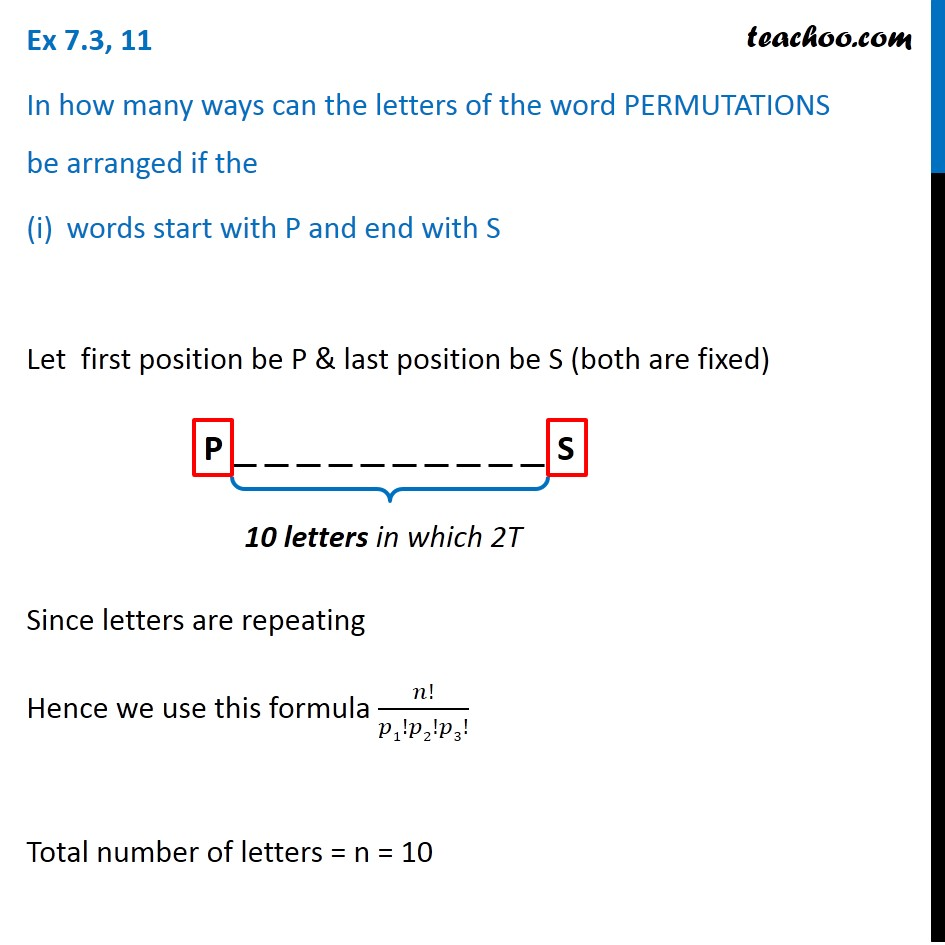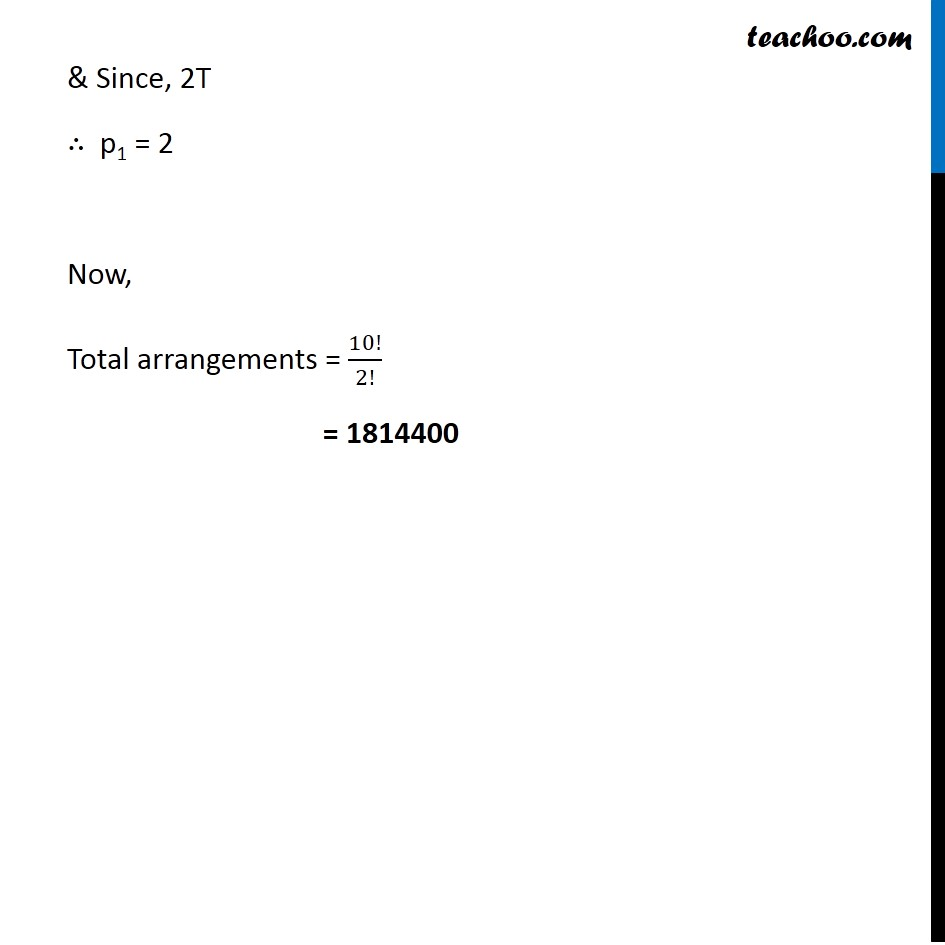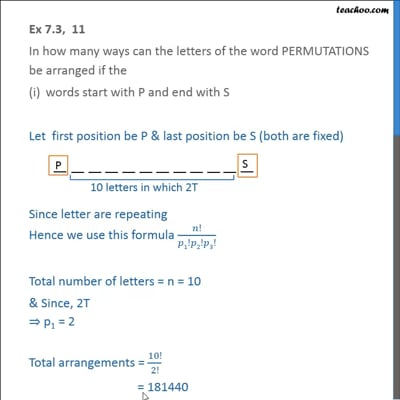This video is only available for Teachoo black users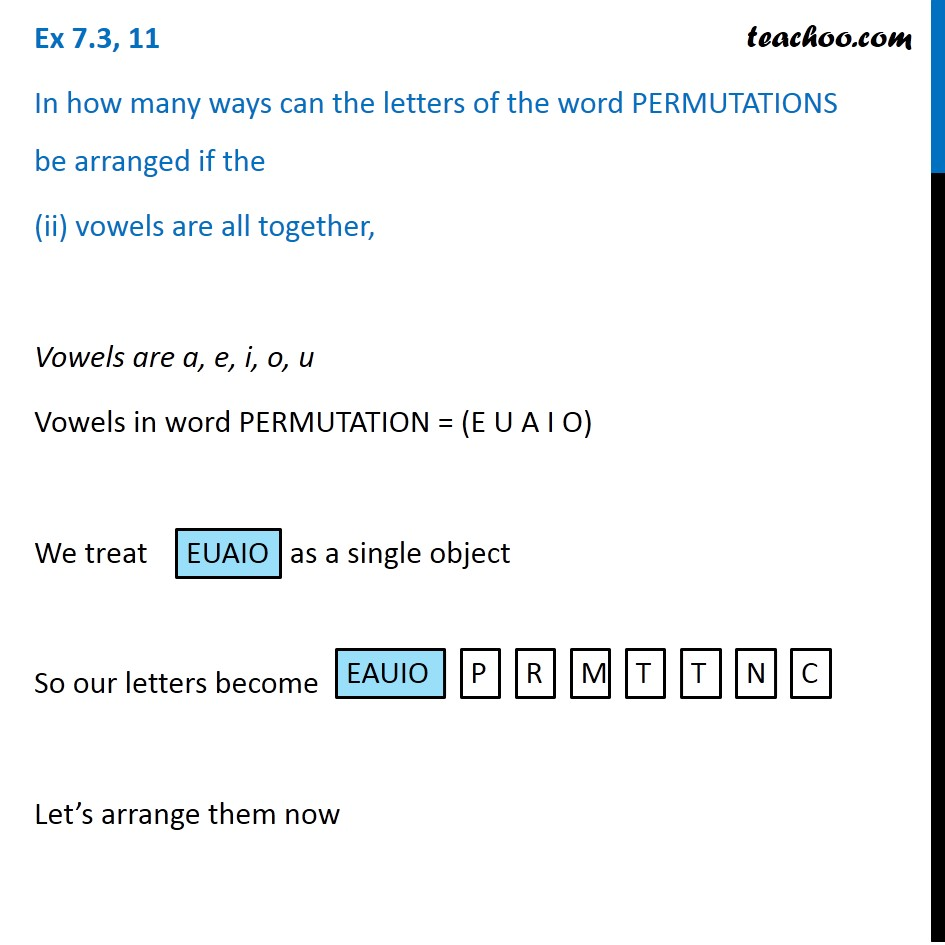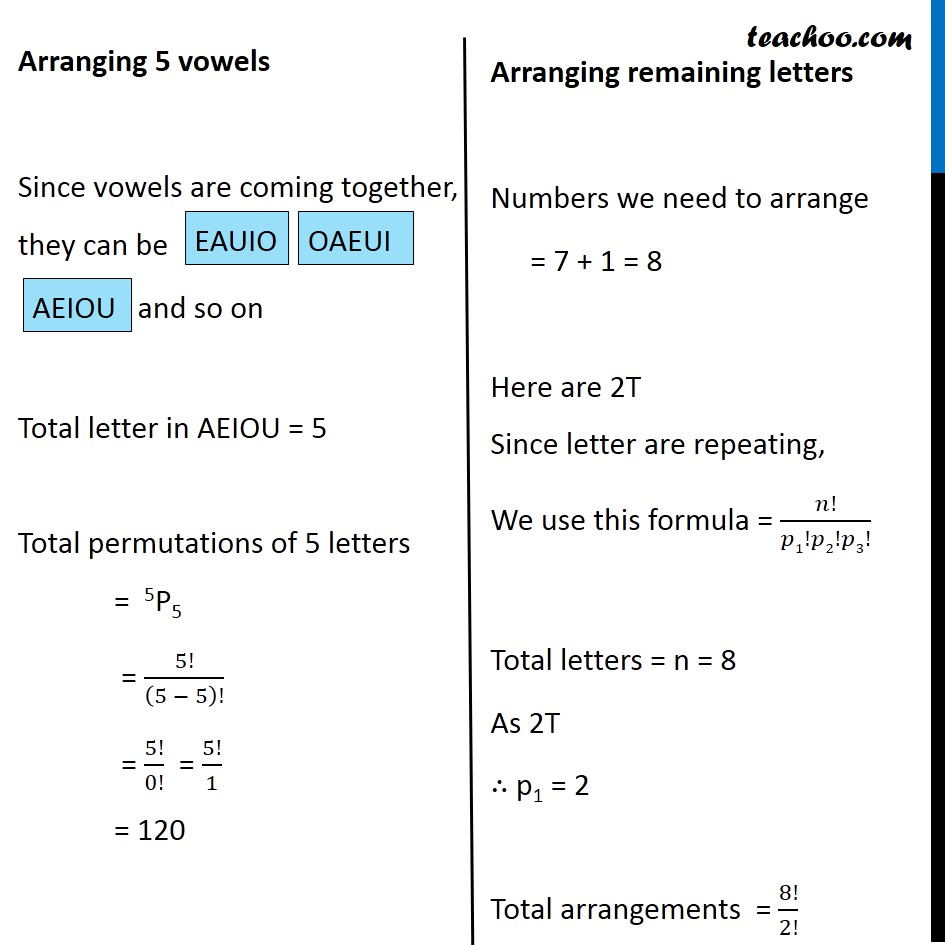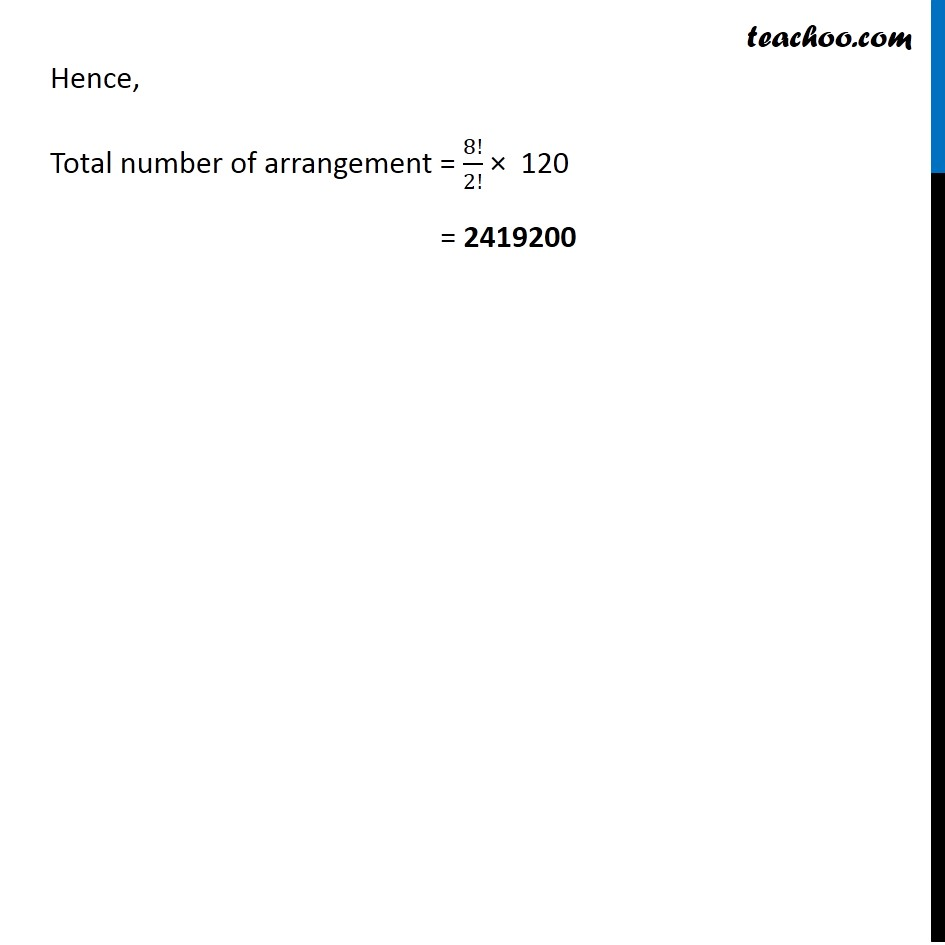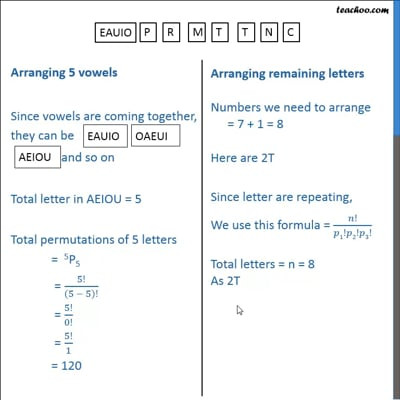This video is only available for Teachoo black users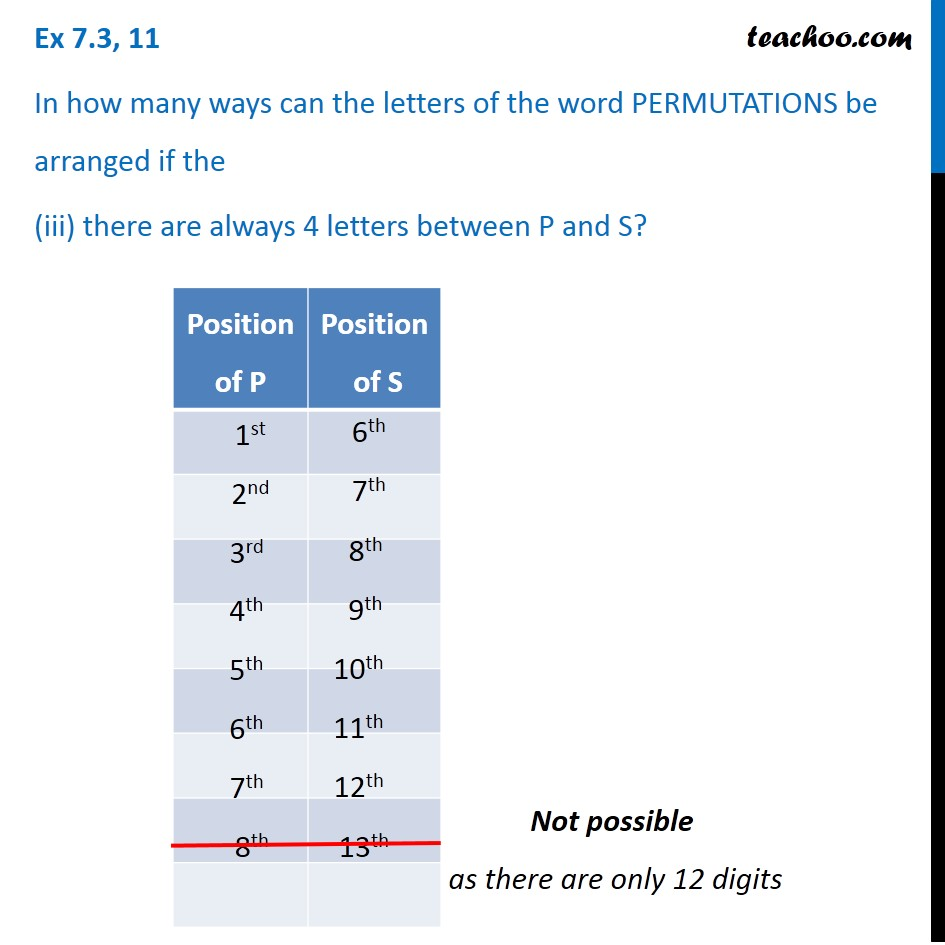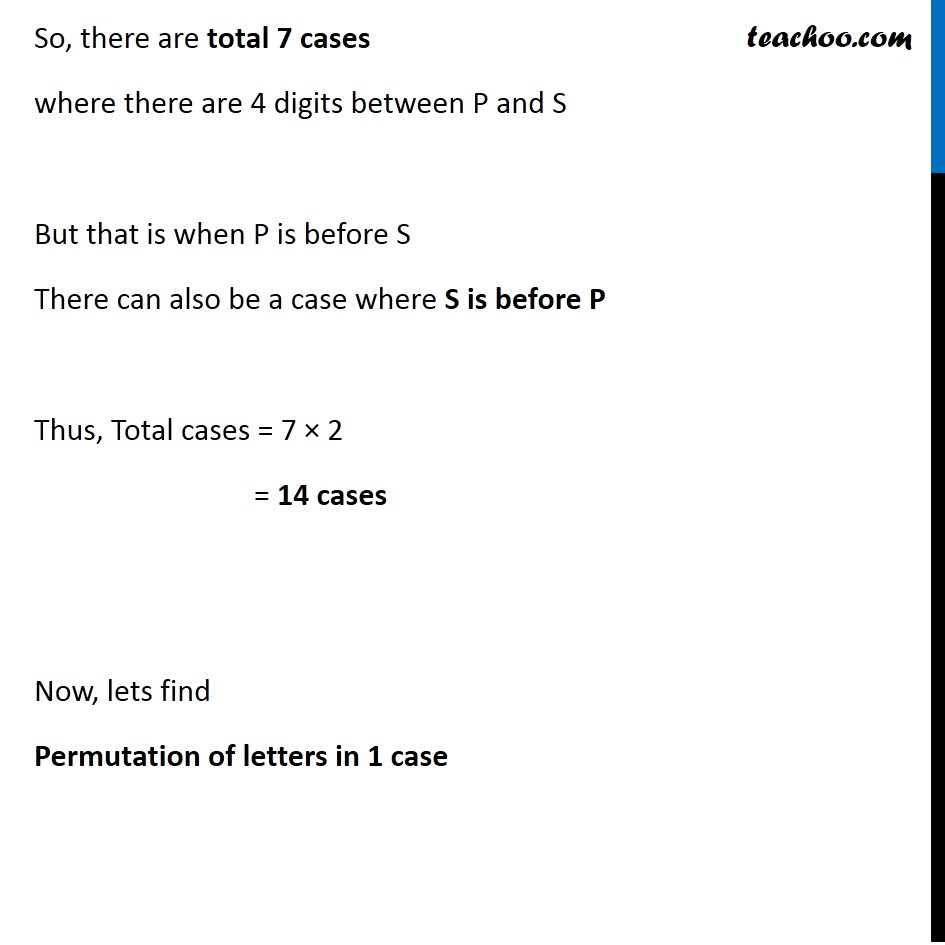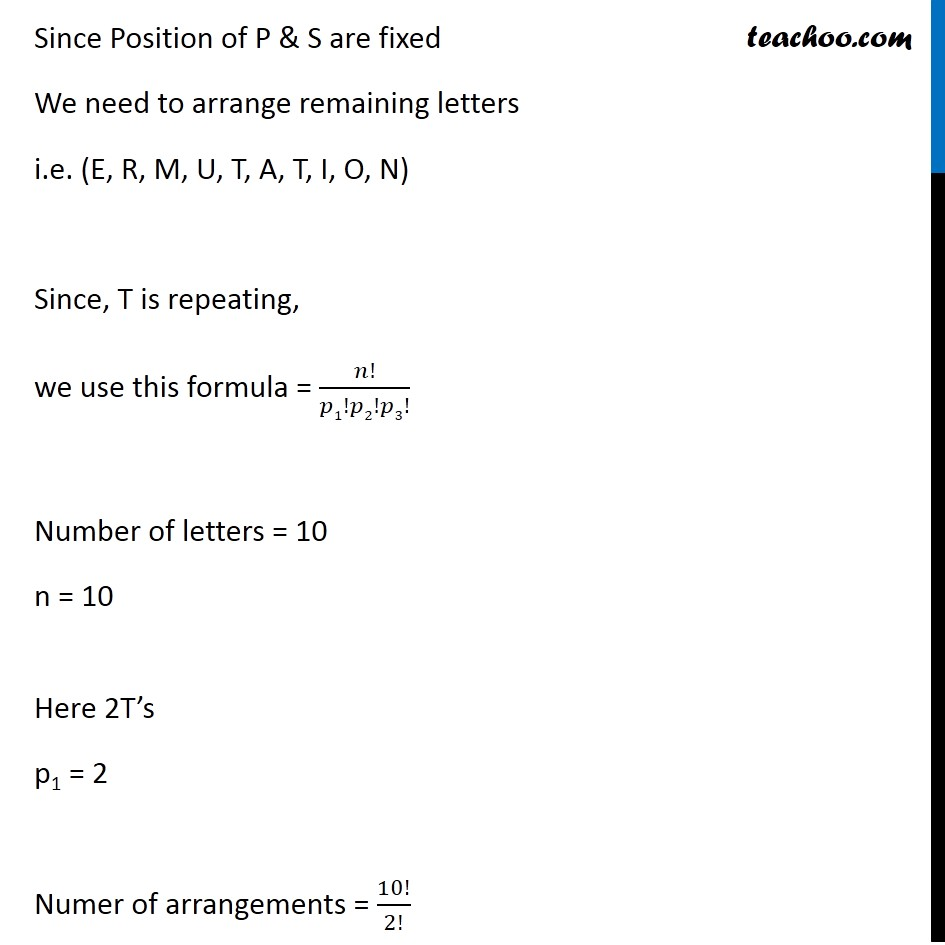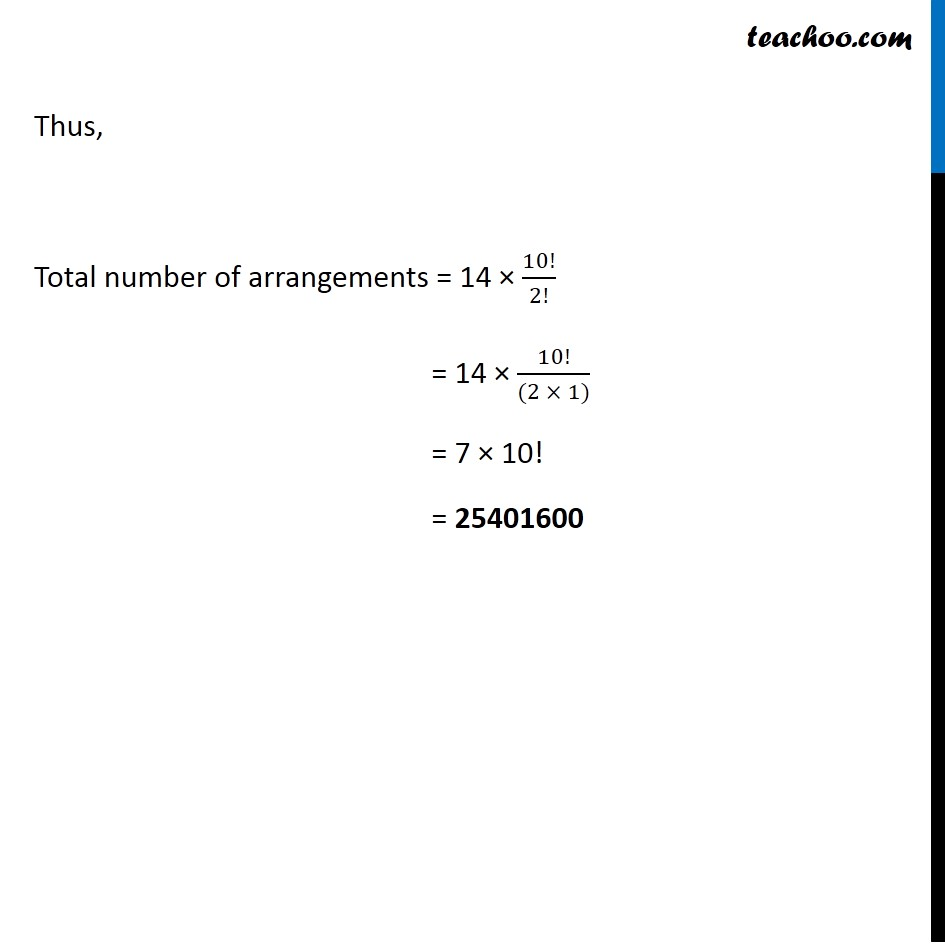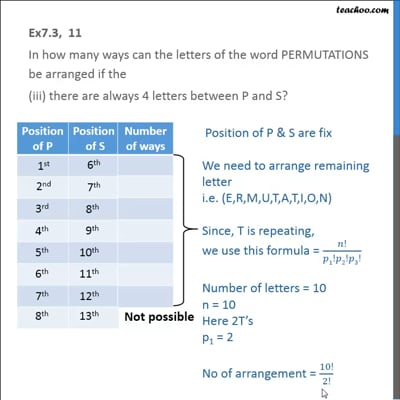This video is only available for Teachoo black users

### Transcript

Ex 7.3, 11 In how many ways can the letters of the word PERMUTATIONS be arranged if the words start with P and end with S Let first position be P & last position be S (both are fixed) Since letters are repeating Hence we use this formula 𝑛!/𝑝1!𝑝2!𝑝3! Total number of letters = n = 10 & Since, 2T ∴ p1 = 2 Now, Total arrangements = 10!/2! = 1814400 Ex 7.3, 11 In how many ways can the letters of the word PERMUTATIONS be arranged if the (ii) vowels are all together, Vowels are a, e, i, o, u Vowels in word PERMUTATION = (E U A I O) We treat as a single object So our letters become Let’s arrange them now Arranging 5 vowels Since vowels are coming together, they can be and so on Total letter in AEIOU = 5 Total permutations of 5 letters = 5P5 = 5!/(5 − 5)! = 5!/0! = 5!/1 = 120 Arranging remaining letters Numbers we need to arrange = 7 + 1 = 8 Here are 2T Since letter are repeating, We use this formula = 𝑛!/𝑝1!𝑝2!𝑝3! Total letters = n = 8 As 2T ∴ p1 = 2 Total arrangements = 8!/2! Hence, Total number of arrangement = 8!/2! × 120 = 2419200 Ex 7.3, 11 In how many ways can the letters of the word PERMUTATIONS be arranged if the (iii) there are always 4 letters between P and S? Not possible as there are only 12 digits So, there are total 7 cases where there are 4 digits between P and S But that is when P is before S There can also be a case where S is before P Thus, Total cases = 7 × 2 = 14 cases Now, lets find Permutation of letters in 1 case Since Position of P & S are fixed We need to arrange remaining letters i.e. (E, R, M, U, T, A, T, I, O, N) Since, T is repeating, we use this formula = 𝑛!/𝑝1!𝑝2!𝑝3! Number of letters = 10 n = 10 Here 2T’s p1 = 2 Numer of arrangements = 10!/2! Thus, Total number of arrangements = 14 × 10!/2! = 14 × 10!/((2 × 1)) = 7 × 10! = 25401600